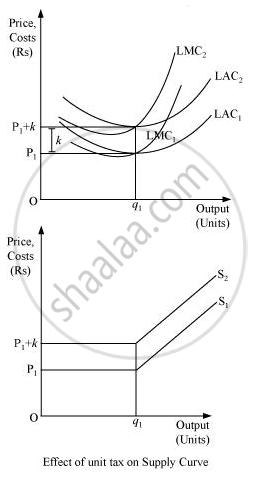Advertisement Remove all ads

# How Does the Imposition of a Unit Tax Affect the Supply Curve of a Firm? - Economics

Short Note

How does the imposition of a unit tax affect the supply curve of a firm?

Advertisement Remove all ads

#### Solution

A unit tax is the tax imposed on per unit of the output sold. Due to the imposition of unit tax, the cost of production per unit of output increases, which ultimately increases the marginal cost. Consequently, the LMC curve will shift leftward upward and as the supply curve is a portion of LMC, so the supply curve will also shift leftward upward.

Let us understand the effect of imposition of unit tax through an example.

Suppose that a firm is facing the price OP1. LAC1 and LMC1 are the long run average cost curve and long run marginal cost curve respectively. Also assume that the government has imposed a unit tax of Rs k per unit of output produced. Now, this will rise the firm’s LAC and LMC, as the firm needs to pay Rs k extra on each output produced. Consequently, LMC1 and LAC1 will shift leftward upwards to LMC2 and LAC2. The magnitude of shift is equal to Rs k. As the supply curve is a part of LMC, it will also shift leftward from S1 to S2, due to the imposition of the tax. Consequently, the firm will now supply lesser units of output.Is there an error in this question or solution?
Advertisement Remove all ads

#### APPEARS IN

NCERT Class 12 Economics Introductory Microeconomics
Chapter 4 The Theory Of The Firm Under Perfect Competition
Exercise | Q 15 | Page 68
Advertisement Remove all ads
Advertisement Remove all ads
Share
Notifications

View all notifications

Forgot password?